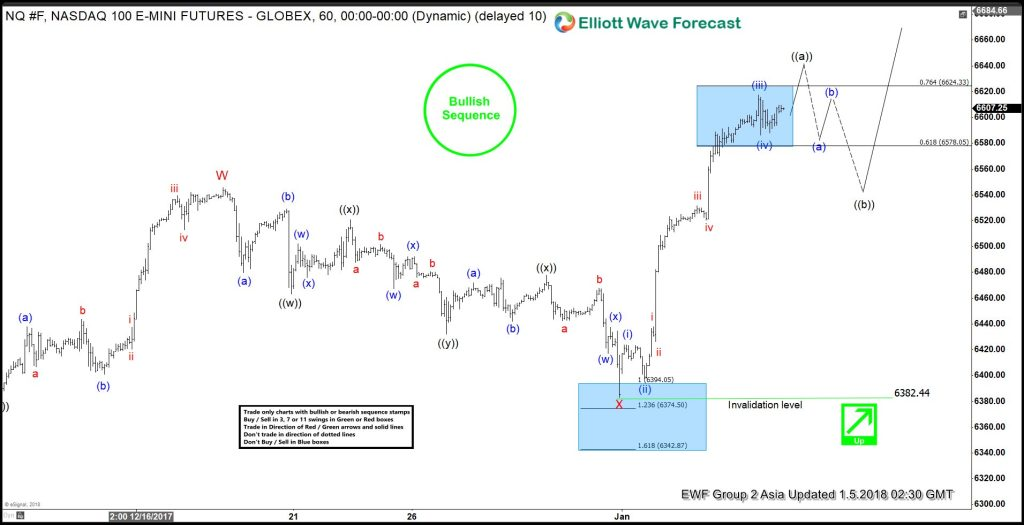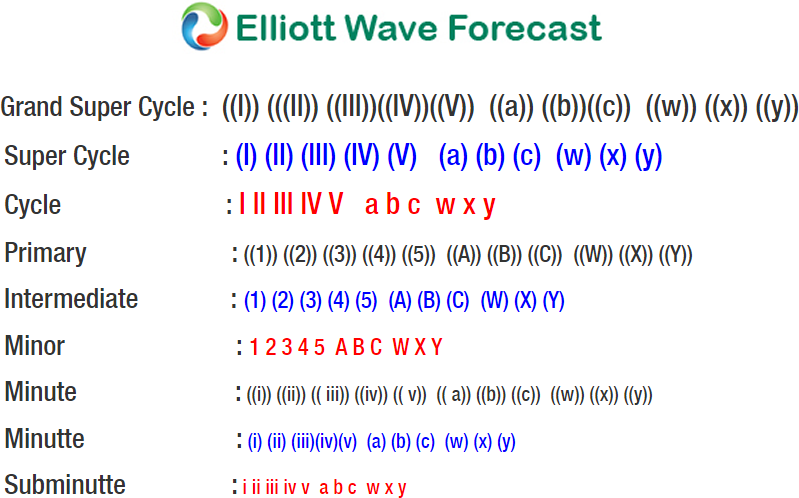# Nasdaq Elliott Wave Analysis: Ending Impulsive Move

Short Term Nasdaq Elliott Wave view suggests that the rally from 12/5 low unfolded as a double three Elliott Wave structure where Minor wave W ended at 6545.75 and Minor wave X ended at 6383.25.  This week, the Index made a new high above Minor wave W at 6545.75, suggesting the next leg higher has started. Internal of Minor wave W unfolded as a double three Elliott Wave structure where Minute wave ((w)) ended at 6427.75, Minute wave ((x)) ended at 6383, and Minute wave ((y)) of W ended at 6545.75.

Internal of Minor wave X pullback unfolded as a triple three Elliott Wave structure where Minute wave ((w)) ended at 6463.25, Minute wave ((x)) ended at 6520.75, Minute wave ((y)) ended at 6432.25, and second Minute wave ((x)) of X ended at 6382.44. Near term, rally from 12/30 low (6383.25) is unfolding as an impulse Elliott Wave structure and could see more upside to end 5 waves up in Minute wave ((a)). Afterwards, the Index should pullback in Minute wave ((b)) in 3, 7, or 11 swing to correct cycle from 12/30 low (6382.44) before the rally resumes. We don’t like selling the Index and while Minute wave ((b)) dips stay above 6382.44, expect Index to extend higher.

#### Nasdaq 1 Hour Elliott Wave ChartSource : https://elliottwave-forecast.com/stock-market/nasdaq-elliott-wave-ending-impulsive-move/